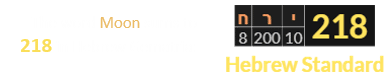Hi, Guest!
Become a Member for an ad-free experience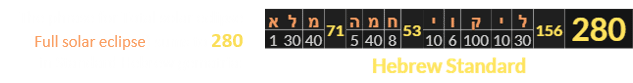280 has matching 192 gematria with Eclipse, which in turn sums back around to 2080.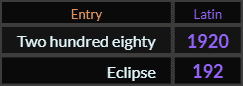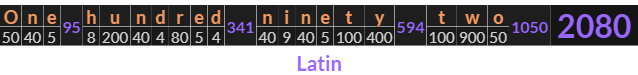### 28 and 33

In numerology, 280 and 2080 both represent 28, the second perfect number. Twenty-eight has matching Ordinal gematria with Thirty-three, which is the Reduction value of Eclipse. It takes 33 solar years for the calendar to fall back in sync with the lunar calendar.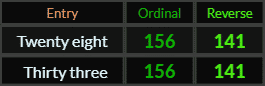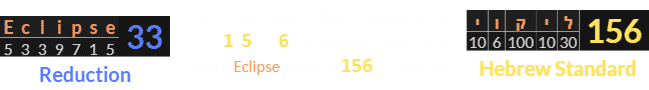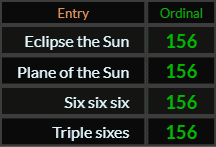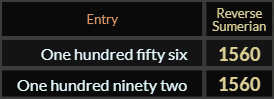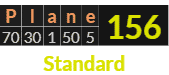### Planes allegedly struck the Twin Towers on 9/11 The 156th Prime number is 911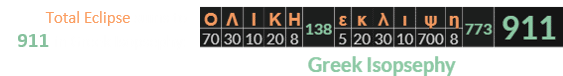Notice how Six six six and Triple sixes both tie in. The Earth, whose orbital velocity is ~66,600 miles per hour, has a polar axis that’s tilted 66.6° from the Plane of the sun: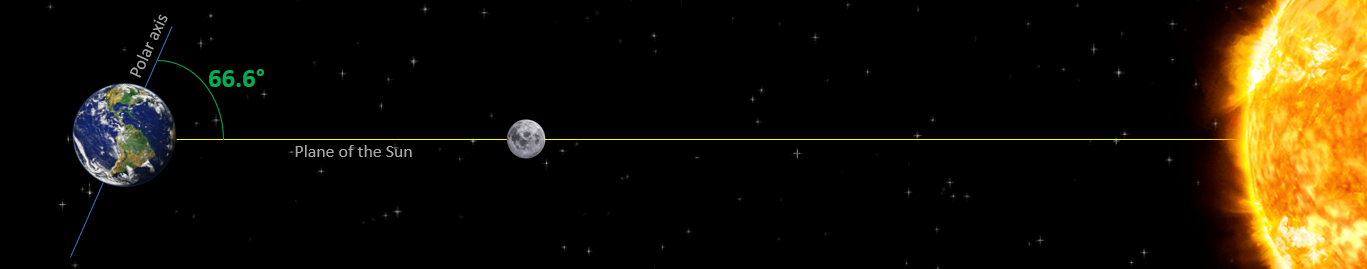### 666 / Pi

In Reduction, Thirty-three connects us to the 666 code.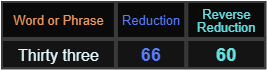An eclipse occurs when the light from the Sun is blocked by a 2nd object from reaching a 3rd. 2÷3 = 0.666. Sun-Moon sums to both 33 and 666.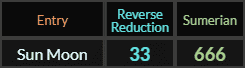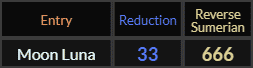Check out this presentation that highlights why 666 is the primary number connected to The Moon.### 666, or 36‘s, is the 36th Triangular number A circle is comprised of 360 total degrees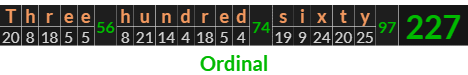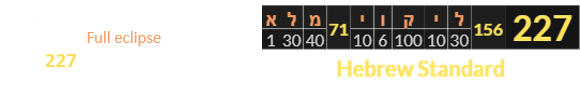This post started with the number 28. The number of days in exactly 28 years is 10,227. The mathematics of the circle are resolved with Pi, which is typically rounded off to 3.14. The smallest equation you can use to get this estimate is 22 ÷ 7.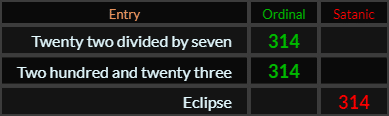The astrological sign ruled by the Moon is Cancer, whose symbol is a sideways 69: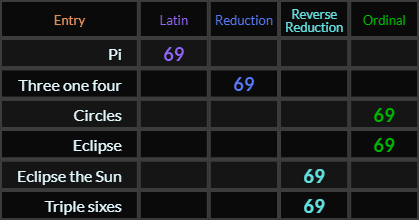### Saros / Metonic Cycles / Tarot### 223 is the 48th Prime number The Latin name for the Moon is Luna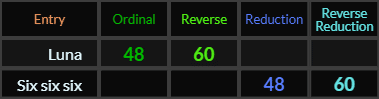6+6+6= 18. The Moon’s Saros cycle is 223 lunar phases in length, which is just a few days more than 18 years.

Meanwhile, the Metonic cycle, which syncs the orbits of the Moon and Sun and determines when a 13th month is added to the lunisolar calendar, is almost exactly 19 years long.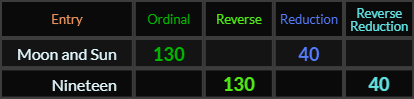In a classical deck of Tarot cards, the Moon is represented by the number 18, while the Sun is represented by the number 19: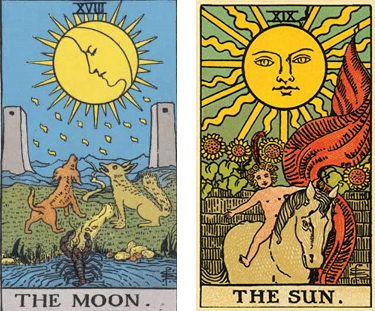Because of the 19-year Metonic cycle, there is regularly a Solar eclipse that falls almost exactly 19 years after a previous one.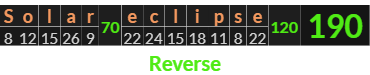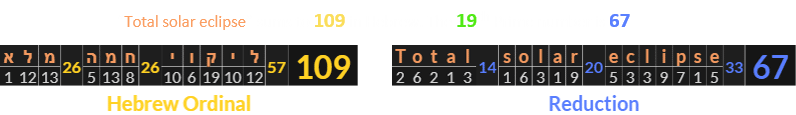“Total solar eclipse” yields a 37 in Hebrew Reduction, which happens to be the sum of the two Tarot cards above.

### 57°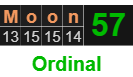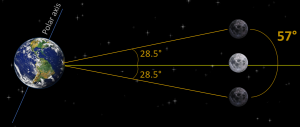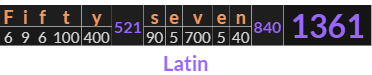### 1361 is the 218th Prime number